# Geometrical shapesPage 1

#### WATCH ALL SLIDES

Slide 1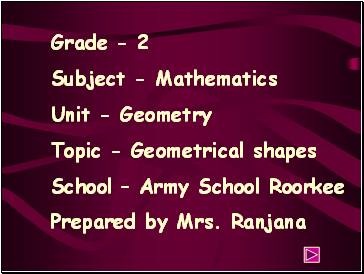Subject - Mathematics

Unit - Geometry

Topic - Geometrical shapes

School – Army School Roorkee

Prepared by Mrs. Ranjana

Slide 2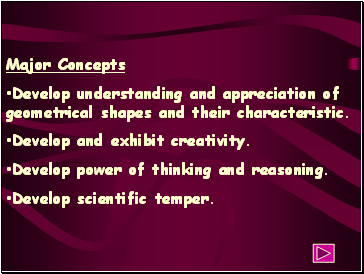## Major Concepts

Develop understanding and appreciation of geometrical shapes and their characteristic.

Develop and exhibit creativity.

Develop power of thinking and reasoning.

Develop scientific temper.

;

;

Slide 3## Minor Concepts

Students will be able to recognized different geometrical shapes.

Students will be able to relate various shapes like that of bangle, cart,cone,box etc with circle,triangle,quadrilateral etc.

Slide 4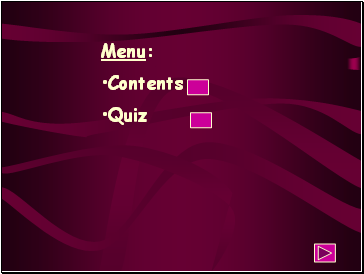Contents

Quiz

Slide 5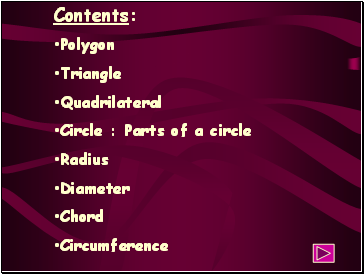Contents:

Polygon

Triangle

Circle : Parts of a circle

Diameter

Chord

Circumference

Slide 6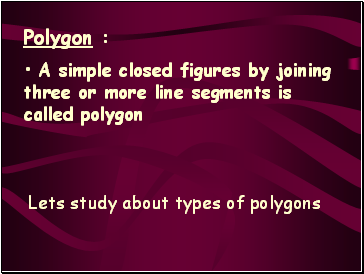## Polygon

A simple closed figures by joining three or more line segments is called polygon

Lets study about types of polygons

Slide 7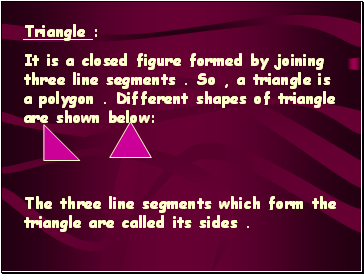## Triangle

It is a closed figure formed by joining three line segments . So , a triangle is a polygon . Different shapes of triangle are shown below:

The three line segments which form the triangle are called its sides .

Slide 8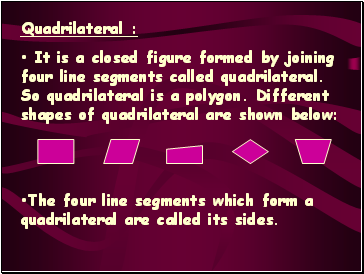It is a closed figure formed by joining four line segments called quadrilateral. So quadrilateral is a polygon. Different shapes of quadrilateral are shown below:

The four line segments which form a quadrilateral are called its sides.

Slide 9## Circle

A closed curve formed in such a way that any point on this curve is equidistant from a fixed point which is in the interior of the curve.

A

D

O

OA = OB = OC = OD

o

B

C

Slide 10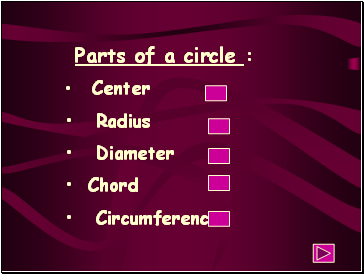## Parts of a circle

Center

Diameter

Chord

Circumference

Slide 11## Center

A fixed point in the interior of circle whose distance from all the points on the circle is same.

A

B

C

D

Go to page:
1  2  3